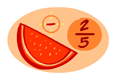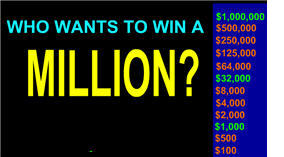# Number and Operations gamesMatch Fraction, decimal, and percentsFraction subtractionNumber line + - integersArithmetic four (all integer operations)Square roots equations